An Introduction to Multivariate Calibration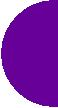Introduction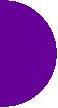This page is based on a seminar I gave to the Microbiology department at University of Wales. It is intended as a gentle introduction to why the multivariate methods used in Chemometrics are preferable to the more traditional methods.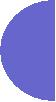Modelling - What and Why?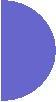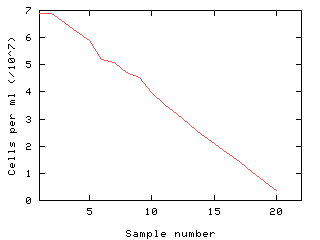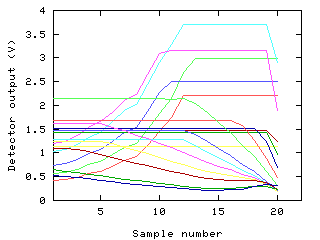In the example above, we wish to measure cell concentration. This is an inconvenient quantity to measure, requiring expensive equipment, or counting by hand, which is time consuming and inaccurate. However, we have a simple instrument which generates many input variables, these bearing some relationship to the cell count. If we had a model relating cell count to these values, we could save much time and money. In the ideal situation, we have a knowledge of the physical processes underlying the situation. A theoretical formula can be used to calculate the cell count from the input variables. For example, physics dictates that if we know the pressure and volume of a known mass of gas, then we can calculate its temperature. In the more common situation, we don't have this sort of information to hand. Possibly the physics of the situation is intractable, or just too difficult to figure out. However, we can see that there is a relationship there. So, how do we handle this? This is where calibration comes in. Rather than trying to figure out the theoretical relationship between input and output variables, we make simple assumptions as to the underlying relationship. Using some given examples of input and output variables, we then try to estimate the parameters of this relationship.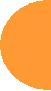Notation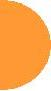Before we move to the basics of calibration, it is worth introducing the basic notation used in the subject.

X variables
The X variables are the quantities we wish to measure in future. Typically, these are more convenient to measure than the values we wish to model.

Y variables
The Y variables are the quantities we wish to predict in future. These will be the values estimated from the X values using the model.

Variance
This is a measure of the spread of a variable about its average value.

Covariance
This is a measure of the similarity of two variables. Variables having high covariance are strongly related to each other. To know the strength of this relationship, we also need to know the variance of the individual variables.

Matrix representation of data
If, for each variable, we measure n samples, and write the measured values as columns of a table, we refer to this table as a matrix. A mathematics of matrices can be defined, such that we can multiply tables by each other, add tables together, etc. Multivariate calibration depends strongly on this representation. For example,

X1X2X3
1 2 3
4 5 6
7 8 9
101112
131415
is represented as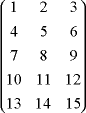in matrix form.

A matrix containing a single column (or row) is known as a column (row) vector.

Matrix transpose
To transpose a matrix, simply write its rows as columns. For example, the transpose of the matrix shown above is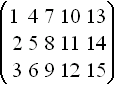The transpose of a matrix is denoted by placing a superscript T, for example XT.Univariate Linear RegressionThis will be familiar to most people as finding the line of best fit through a cloud of points. We assume that the relationship between a single X variable and one Y variable is linear. i.e. Y = bX + a, where b is the slope of the line, while a is the intercept at the Y axis.

Univariate linear regression estimates the values of b and a by minimising the sum of squared vertical distances from points to the line. In other words, we choose a candidate slope, b and intercept, a. For each recorded (X, Y) pair, we square Y - bX - a and add it to the total. The line having the smallest total is the best fit line.

In practise, calculus gives us a formula for estimating b directly, and thence a, as follows: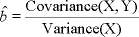The ^ indicates that the value is an estimate of b.

We can ignore a if we centre all our variables before using them. To centre each variable, we merely calculate its average value, then subtract this value from all sample values. a can be calculated after modelling using the estimated value of b and the subtracted averages.

When working with centred data, we can express the linear regression equation for b in matrix form as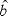=(XTX)-1XTY.

Note that if the variance of X is zero, then we cannot estimate b. This occurs when the X variable has the same value for all values of Y.

## Example: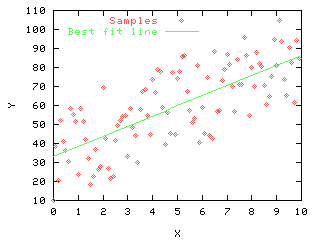The graph shows the best fit line through a data set. The estimated slope is 5.35, with intercept 33.02.Multiple Linear RegressionWhat if we have more than one X variable? Again, we can assume a linear relationship and form a linear regression. In this case, we have Y = b1x1 + b2x2 + ... b3x3 + bnxn

As it happens, the matrix form of the linear regression equation,=(XTX)-1XTY also works for multiple X variables. In this case, the resulting estimate of b is a vector containing the weights applied to the X variables.

In the case of multiple linear regression, we have to be a little more careful. There are many situations when (XTX)-1 cannot be calculated.

## For example:

 X =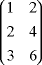XTX =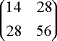(XTX)-1 =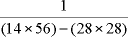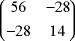=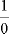We end up trying to divide by zero. This situation will arise whenever a (non-zero) weighted sum of the X variables gives a zero result, or one of the rows or columns of X contains all zeros. In the example above, X2 - 2X1=0. When such a weighted sum exists, the X variables involved are said to be collinear.

In practice, it is rare for us to be able to measure variables with absolute accuracy. So, even when some of the X variables are actually collinear, our experimental values will not show this. So, what happens when some of the X variables are nearly collinear?

First, an example where this is the case: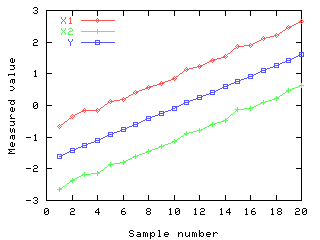Here, we have two nearly collinear X variables and wish to form a model on the Y variable. After centring the variables, we have:

 (XTX)-1 =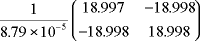=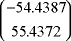The model generated calculates the prediction using Y = 55.4 X2 - 54.4 X1 + 1. This looks a little strange. It's predicting Y as a difference between the variables, rather than an average, which looks more sensible. What happens when we try to predict some new Y values from new X measurements?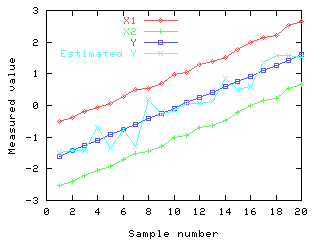The prediction is useless! Even though both X variables obviously give information about Y, the prediction derived from this model is bad. So, what went wrong?

Looking back at the value of (XTX)-1, we see that the matrix is multiplied by the inverse of a very small number. It turns out that this small number is very sensitive to noise in the measured X variables. As the X variables become more and more collinear, the value tends to zero. Small changes in collinearity alter this value radically. In this particular case, the effect of this on the model is particularly bad, because the model tends to amplify noise in the variables.

Note that if the number of recorded samples is less than the number of X variables, then collinearity is guaranteed to occur. In this situation, the usual solution is to discard variables. The process of selecting variables for MLR is known as Stepwise Multiple Linear Regression.

## Summary of the properties of MLR

• It can't handle collinearity.
• It is unstable with near collinearity.
• Relevant variables have to be discarded to avoid these problems.Principal Components AnalysisIn the previous section, we saw that MLR is unstable when there are correlated X variables. This gives a good example of why we need to examine the structure within data sets, rather than using them blindly. Finding such structure by hand can be extremely difficult, even in relatively simple cases.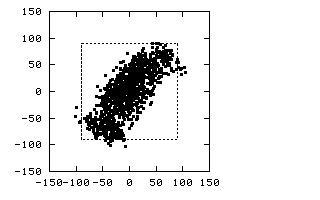The above picture shows such a case. Click on the image to see the animation (208K). The data set consists of a cluster of values in three variables. Plotting any two of the variables against each other doesn't show any obvious structure. However, when we rotate the data set, we see that the set is actually very structured. So, for a simple, 3-d set, it can be hard to see the structure. Imagine the problem when 300 variables are being used! Principal components analysis provides a method for finding structure in such data sets. Put simply, rotates the data into a new set of axes, such that the first few axes reflect most of the variations within the data. By plotting the data on these axes, we can spot major underlying structures automatically. The value of each point, when rotated to a given axis, is called the principal component value. For example, the plot below shows the first two principal components of the data set used in the animation: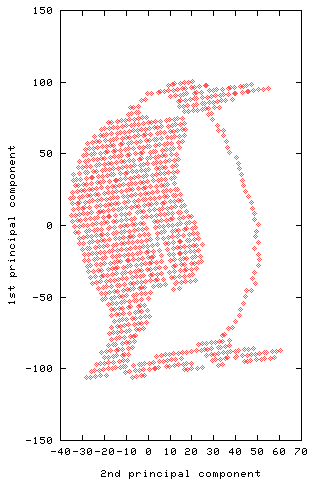Principal Components RegressionPrincipal Components Analysis selects a new set of axes for the data These are selected in decreasing order of variance within the data They are also (of course) perpendicular to each other Hence the principal components are uncorrelated Some components may be constant, but these will be among the last selected. The problem noted with MLR was that correlated variables cause instability. So, how about calculating principal components, throwing away the ones which only appear to contribute noise (or constants), and using MLR on these? This process gives the modelling method known as Principal Components Regression. Rather than forming a single model, as we did with MLR, we can now form models using 1, 2, ... components, and decide how many components are optimal. If the original variables contained collinearity, then some of our components will contribute only noise. So long as we drop these, we can guarantee that our models will be stable. For example, going back to the data presented in the description of MLR. First we calculate the principal components: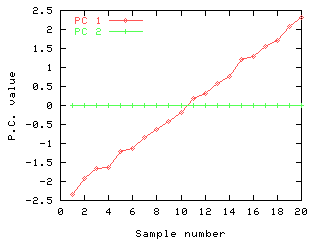The first principal components shows the major variation within the two X variables, this being the one which is related to the Y variable. The second component contains the small noise factor which is responsible for the differences between the X variables. We can see quite clearly that the second component is not contributing anything useful, so can drop this one for the MLR step: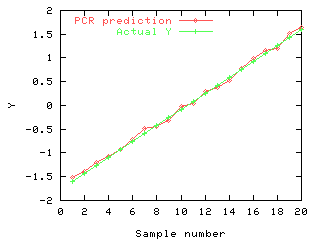This graph shows the predicted and actual values of Y for the test data set, using PCR. Dropping the second component has improved the predictions significantly. The model generated by PCR actually calculates the mean of the X variables, rather than the contrast generated by MLR. This seems a much more sensible proposition.Partial Least Squares RegressionThe intention, in using PCR, was to extract the underlying effects in the X data, and to use these to predict the Y values. In this way, we could guarantee that only independent effects were used, and that low-variance noise effects were excluded. This improved the quality of the model significantly. However, PCR still has a problem: if the relevant underlying effects are small in comparison with some irrelevant ones, then they may not appear among the first few principal components. So, we are still left with a component selection problem - we cannot just include the first n principal components, as these may serve to degrade the performance of the model. Instead, we have to extract all components, and determine whether adding each one of these improves the model. This is a complex problem. Partial Least Squares Regression (PLSR) solves the problem. The algorithm used examines both X and Y data and extracts components (now called factors), which are directly relevant to both sets of variables. These are extracted in decreasing order of relevance. So, to form a model now, all we have to do is extract the correct number of factors to model relevant underlying effects.Latent Variables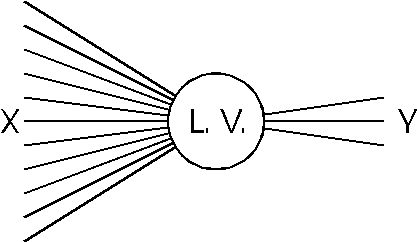Throughout this page, we have talked about "underlying effects". In multivariate calibration, these are called "latent variables". In other words, a latent variable is one which we do not observe directly, but we can infer its existance by the properties of our observed variables. We can view latent variables in several ways: Assuming that all relationships between latent and observed variables are linear, we can use PCA (if we assume that only the X variables are affected by the latent variables), or PLSR (assuming that both X and Y are affected). If the relationships are thought to be non-linear, then PCA and PLSR are not ideal, since these assume linearity. If we have an idea of the mathematical form of the nonlinearity, we can try transforming the X and Y variables to linearise them. Failing that, we can use Artificial Neural Networks (ANNs), which use a latent variable model which does not assume linearity.Group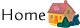Back to the group's homepage.